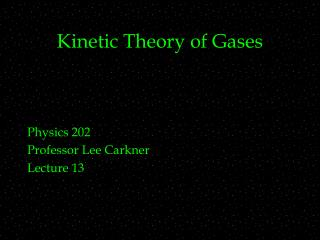DownloadDownload PresentationKinetic Theory of Gases

# Kinetic Theory of Gases

Télécharger la présentation## Kinetic Theory of Gases

- - - - - - - - - - - - - - - - - - - - - - - - - - - E N D - - - - - - - - - - - - - - - - - - - - - - - - - - -
##### Presentation Transcript

1. Kinetic Theory of Gases Physics 202 Professor Lee Carkner Lecture 13

2. What is a Gas? • But where do pressure and temperature come from? • A gas is made up of molecules (or atoms) • The pressure is a measure of the force the molecules exert when bouncing off a surface • We need to know something about the microscopic properties of a gas to understand its behavior

3. Mole • A gas is composed of molecules • m = • N = • When thinking about molecules it sometimes is helpful to use the mole 1 mol = 6.02 X 1023 molecules • 6.02 x 1023 is called Avogadro’s number (NA) • M = M = mNA • A mole of any gas occupies about the same volume

4. Ideal Gas • Specifically, 1 mole of any gas held at constant temperature and constant volume will have almost the same pressure • Gases that obey this relation are called ideal gases • A fairly good approximation to real gases

5. Ideal Gas Law • The temperature, pressure and volume of an ideal gas is given by: pV = nRT • Where: • R is the gas constant 8.31 J/mol K • V in cubic meters

6. Work and the Ideal Gas Law p=nRT (1/V)

7. Isothermal Process • If we hold the temperature constant in the work equation: W = nRT ln(Vf/Vi) • Work for ideal gas in isothermal process

8. Isotherms • From the ideal gas law we can get an expression for the temperature • For an isothermal process temperature is constant so: • If P goes up, V must go down • Lines of constant temperature • One distinct line for each temperature

9. Constant Volume or Pressure W=0 W = pdV = p(Vf-Vi) W = pDV • For situations where T, V or P are not constant, we must solve the integral • The above equations are not universal

10. Gas Speed • The molecules bounce around inside a box and exert a pressure on the walls via collisions • The pressure is a force and so is related to velocity by Newton’s second law F=d(mv)/dt • The rate of momentum transfer depends on volume • The final result is: p = (nMv2rms)/(3V) • Where M is the molar mass (mass of 1 mole)

11. RMS Speed • There is a range of velocities given by the Maxwellian velocity distribution • We take as a typical value the root-mean-squared velocity (vrms) • We can find an expression for vrms from the pressure and ideal gas equations vrms = (3RT/M)½ • For a given type of gas, velocity depends only on temperature

12. Maxwell’sDistribution

13. Translational Kinetic Energy • Using the rms speed yields: Kave = ½mvrms2 Kave = (3/2)kT • Where k = (R/NA) = 1.38 X 10-23 J/K and is called the Boltzmann constant • Temperature is a measure of the average kinetic energy of a gas

14. Maxwellian Distribution and the Sun • The vrms of protons is not large enough for them to combine in hydrogen fusion • There are enough protons in the high-speed tail of the distribution for fusion to occur

15. Next Time • Read: 19.8-19.11